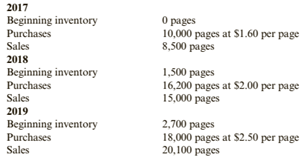Chapter 6, Problem 55E### Cornerstones of Financial Accounti...

4th Edition
Jay Rich + 1 other
ISBN: 9781337690881

#### Solutions

Chapter
Section### Cornerstones of Financial Accounti...

4th Edition
Jay Rich + 1 other
ISBN: 9781337690881
Textbook Problem
5 views

# Effects of FIFO and LIFOSheepskin Company sells to colleges and universities a special paper that is used for diplomas. Sheepskin typically makes one purchase of the special paper each year on January 1. Assume that Sheepskin uses a perpetual inventory system. You have the following data for the 3 years ending in 2019:Required:1. What would the ending inventory and cost of goods sold be for each year if FIFO is used?2. What would the ending inventory and cost of goods sold be for each year if LIFO is used?3. CONCEPTUAL CONNECTION For each year, explain the cause of the differences in cost of goods sold under FIFO and LIFO.

To determine

(a)

Inventory costing methods:

FIFO and LIFO are those methods which are used for calculation of closing inventory and cost of goods sold.

The cost of ending inventory and the cost of goods sold using the FIFO.

Explanation

The given information is as follows:

Total available units are:

In the year 2017 :

Purchases=10000 units @ $1.60 each Sales=8500 pages Closing inventory =Beginning inventory + PurchaseSales=0+100008500=1500 pages In the year 2018 : Opening Inventory =1500 pages @$1.60 each

Purchases=16200 units @ $2 each Sales=15000 pages Closing inventory =Beginning inventory + PurchaseSales=1500+1620015000=2700 pages In the year 2019 : Opening Inventory =2700 pages @$2 each

Purchases=18000 units @ \$2.5 each

Sales=20100 pages

Closing inventory =Beginning inventory + PurchaseSales=2700+1800020100=600 pages

Calculation of Closing Inventory as per FIFO Method:

Under this method, which material purchased first, issued first for production

To determine

(b)

Inventory costing methods:

FIFO and LIFO are those methods which are used for calculation of closing inventory and cost of goods sold.

The cost of ending inventory and the cost of goods sold using the LIFO.

To determine

(c)

Inventory costing methods:

FIFO and LIFO are those methods which are used for calculation of closing inventory and cost of goods sold.

The reason for difference in cost of goods sold under both methods.

### Still sussing out bartleby?

Check out a sample textbook solution.

See a sample solution

#### The Solution to Your Study Problems

Bartleby provides explanations to thousands of textbook problems written by our experts, many with advanced degrees!

Get Started